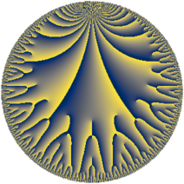# Properties

 Label 48.22.jLevel $48$ Weight $22$ Character orbit 48.j Rep. character $\chi_{48}(13,\cdot)$ Character field $\Q(\zeta_{4})$ Dimension $168$ Sturm bound $176$

# Related objects

## Defining parameters

 Level: $$N$$ $$=$$ $$48 = 2^{4} \cdot 3$$ Weight: $$k$$ $$=$$ $$22$$ Character orbit: $$[\chi]$$ $$=$$ 48.j (of order $$4$$ and degree $$2$$) Character conductor: $$\operatorname{cond}(\chi)$$ $$=$$ $$16$$ Character field: $$\Q(i)$$ Sturm bound: $$176$$

## Dimensions

The following table gives the dimensions of various subspaces of $$M_{22}(48, [\chi])$$.

Total New Old
Modular forms 340 168 172
Cusp forms 332 168 164
Eisenstein series 8 0 8

## Trace form

 $$168 q - 3208660 q^{4} + 10875501588 q^{8} + O(q^{10})$$ $$168 q - 3208660 q^{4} + 10875501588 q^{8} + 141333304008 q^{10} - 134666641480 q^{11} - 549415987992 q^{12} - 7580676743484 q^{14} - 4613203125000 q^{15} + 4557010413696 q^{16} + 4002828492348 q^{18} + 46007763621432 q^{19} + 196422031250000 q^{20} + 223201549491712 q^{22} + 498907865821284 q^{24} - 753312645084980 q^{26} + 8468084858206952 q^{28} + 4866346900433200 q^{29} - 1422261970204248 q^{30} + 19671078887539224 q^{31} - 10070930361655680 q^{32} + 47180565540051200 q^{34} + 40414305744882264 q^{35} - 8452258277913684 q^{36} + 14491417054238768 q^{37} + 175187804522404712 q^{38} + 32650532815240976 q^{40} - 67227589302091020 q^{42} - 76769615939711560 q^{43} - 182643993443571464 q^{44} + 948354398771348776 q^{46} + 889326175522911984 q^{48} - 13405100737998816168 q^{49} - 1465068799295972508 q^{50} - 886367974041189432 q^{51} + 4746491333968909104 q^{52} - 3779975358420295216 q^{53} + 312130956255487884 q^{54} - 8134614321801781632 q^{56} + 6043369462577228432 q^{58} + 12377493140660983072 q^{59} - 8593413961664790216 q^{60} - 10385362452353654064 q^{61} + 37423926077749156092 q^{62} + 7879442335054326792 q^{63} + 4792955955269992328 q^{64} + 18728322261145077424 q^{65} - 27808549328340060696 q^{66} + 6896811892292349552 q^{67} - 88677994614761171936 q^{68} + 3951842806031913936 q^{69} + 38382149352744138848 q^{70} + 22875656474550291804 q^{72} + 52823343512087181932 q^{74} - 116704571203614486384 q^{75} - 222572314827858182296 q^{76} + 166317786761690297552 q^{77} - 199847847924366199236 q^{78} - 393813732471461428504 q^{79} + 598479047524721480968 q^{80} - 2042487797121564038568 q^{81} - 962621930555632650920 q^{82} + 40962170285742727960 q^{83} + 405105066241430891400 q^{84} - 323657194960781250000 q^{85} - 1133736219623245285424 q^{86} - 1685594173511234015712 q^{88} + 277262537316581571912 q^{90} - 25315851195664044872 q^{91} - 4114711634977220502992 q^{92} + 3028913342720877586520 q^{94} - 1009256133734524738608 q^{95} + 3008416547990561109120 q^{96} - 9484196106654323201240 q^{98} - 469553544847523553480 q^{99} + O(q^{100})$$

## Decomposition of $$S_{22}^{\mathrm{new}}(48, [\chi])$$ into newform subspaces

The newforms in this space have not yet been added to the LMFDB.

## Decomposition of $$S_{22}^{\mathrm{old}}(48, [\chi])$$ into lower level spaces

$$S_{22}^{\mathrm{old}}(48, [\chi]) \cong$$ $$S_{22}^{\mathrm{new}}(16, [\chi])$$$$^{\oplus 2}$$